xFirst law of thermodynamicsOverview

The first law of thermodynamics
Thermodynamics
Thermodynamics is a physical science that studies the effects on material bodies, and on radiation in regions of space, of transfer of heat and of work done on or by the bodies or radiation...

is an expression of the principle of conservation of work.

The law states that energy
Energy
In physics, energy is an indirectly observed quantity. It is often understood as the ability a physical system has to do work on other physical systems...

can be transformed, i.e. changed from one form to another, but cannot be created nor destroyed. It is usually formulated by stating that the change in the internal energy
Internal energy
In thermodynamics, the internal energy is the total energy contained by a thermodynamic system. It is the energy needed to create the system, but excludes the energy to displace the system's surroundings, any energy associated with a move as a whole, or due to external force fields. Internal...

of a system is equal to the amount of heat
Heat
In physics and thermodynamics, heat is energy transferred from one body, region, or thermodynamic system to another due to thermal contact or thermal radiation when the systems are at different temperatures. It is often described as one of the fundamental processes of energy transfer between...

supplied to the system, minus the amount of work performed by the system on its surroundings.
The first explicit statement of the first law of thermodynamics, by Rudolf Clausius
Rudolf Clausius
Rudolf Julius Emanuel Clausius , was a German physicist and mathematician and is considered one of the central founders of the science of thermodynamics. By his restatement of Sadi Carnot's principle known as the Carnot cycle, he put the theory of heat on a truer and sounder basis...

in 1850, referred to cyclic thermodynamic processes.
"In all cases in which work is produced by the agency of heat, a quantity of heat is consumed which is proportional to the work done; and conversely, by the expenditure of an equal quantity of work an equal quantity of heat is produced."

Clausius stated the law also in another form, this time referring to the existence of a function of state of the system called the internal energy, and expressing himself in terms of a differential equation for the increments of a thermodynamic process.Unanswered QuestionsDiscussionsIdeal Gas lawsEncyclopedia
The first law of thermodynamics
Thermodynamics
Thermodynamics is a physical science that studies the effects on material bodies, and on radiation in regions of space, of transfer of heat and of work done on or by the bodies or radiation...

is an expression of the principle of conservation of work.

The law states that energy
Energy
In physics, energy is an indirectly observed quantity. It is often understood as the ability a physical system has to do work on other physical systems...

can be transformed, i.e. changed from one form to another, but cannot be created nor destroyed. It is usually formulated by stating that the change in the internal energy
Internal energy
In thermodynamics, the internal energy is the total energy contained by a thermodynamic system. It is the energy needed to create the system, but excludes the energy to displace the system's surroundings, any energy associated with a move as a whole, or due to external force fields. Internal...

of a system is equal to the amount of heat
Heat
In physics and thermodynamics, heat is energy transferred from one body, region, or thermodynamic system to another due to thermal contact or thermal radiation when the systems are at different temperatures. It is often described as one of the fundamental processes of energy transfer between...

supplied to the system, minus the amount of work performed by the system on its surroundings.

## Original statements

The first explicit statement of the first law of thermodynamics, by Rudolf Clausius
Rudolf Clausius
Rudolf Julius Emanuel Clausius , was a German physicist and mathematician and is considered one of the central founders of the science of thermodynamics. By his restatement of Sadi Carnot's principle known as the Carnot cycle, he put the theory of heat on a truer and sounder basis...

in 1850, referred to cyclic thermodynamic processes.
"In all cases in which work is produced by the agency of heat, a quantity of heat is consumed which is proportional to the work done; and conversely, by the expenditure of an equal quantity of work an equal quantity of heat is produced."

Clausius stated the law also in another form, this time referring to the existence of a function of state of the system called the internal energy, and expressing himself in terms of a differential equation for the increments of a thermodynamic process. This equation may be translated into words as follows:
In a thermodynamic process, the increment in the internal energy of a system is equal to the difference between the increment of heat accumulated by the system and the increment of work done by it.

## Description

The first law of thermodynamics was expressed in two ways by Clausius. One way referred to cyclic processes and the inputs and outputs of the system, but did not refer to increments in the internal state of the system. The other way referred to any incremental change in the internal state of the system, and did not expect the process to be cyclic. A cyclic process is one which can be repeated indefinitely often and still eventually leave the system in its original state.

In each repetition of a cyclic process, the work done by the system is proportional to the heat consumed by the system. In a cyclic process in which the system does work on its surroundings, it is necessary that some heat be taken in by the system and some be put out, and the difference is the heat consumed by the system in the process. The constant of proportionality is universal and independent of the system and was measured by Joule
James Prescott Joule
James Prescott Joule FRS was an English physicist and brewer, born in Salford, Lancashire. Joule studied the nature of heat, and discovered its relationship to mechanical work . This led to the theory of conservation of energy, which led to the development of the first law of thermodynamics. The...

in 1845 and 1847.

In any incremental process, the change in the internal energy is considered due to a combination of heat
Heat
In physics and thermodynamics, heat is energy transferred from one body, region, or thermodynamic system to another due to thermal contact or thermal radiation when the systems are at different temperatures. It is often described as one of the fundamental processes of energy transfer between...

added to the system and work
Work (thermodynamics)
In thermodynamics, work performed by a system is the energy transferred to another system that is measured by the external generalized mechanical constraints on the system. As such, thermodynamic work is a generalization of the concept of mechanical work in mechanics. Thermodynamic work encompasses...

done by the system. Taking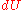as an infinitesimal (differential) change in internal energy, one writes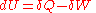where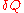and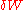are infinitesimal amounts of heat supplied to the system and work done by the system, respectively. Note that the minus sign in front ofindicates that a positive amount of work done by the system leads to energy being lost from the system. (An alternate convention is to consider the work performed on the system by its surroundings. This leads to a change in sign of the work. This is the convention adopted by many modern textbooks of physical chemistry, such as those by Peter Atkins
Peter Atkins
Peter William Atkins is a British chemist and former Professor of Chemistry at the University of Oxford and a Fellow of Lincoln College. He is a prolific writer of popular chemistry textbooks, including Physical Chemistry, Inorganic Chemistry, and Molecular Quantum Mechanics...

and Ira Levine, but many textbooks on physics define work as work done by the system.)

When a system expands in a quasistatic process
Quasistatic process
In thermodynamics, a quasistatic process is a thermodynamic process that happens infinitely slowly. However, it is very important of note that no real process is quasistatic...

, the work done on the environment is the product of pressure (P) and volume (V) change, i.e. , whereas the work done on the system is . The change in internal energy of the system is: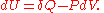Work and heat are expressions of actual physical processes which add or subtract energy, while U is a mathematical abstraction that keeps account of the exchanges of energy that befall the system. Thus the term heat formeans that amount of energy added as the result of heating, rather than referring to a particular form of energy. Likewise, work energy formeans "that amount of energy lost as the result of work". Internal energy is a property of the system whereas work done and heat supplied are not. A significant result of this distinction is that a given internal energy changecan be achieved by, in principle, many combinations of heat and work.

The internal energy of a system is not uniquely defined. It is defined only up to an arbitrary additive constant of integration, which can be adjusted to give arbitrary reference zero levels. This non-uniqueness is in keeping with the abstract mathematical nature of the internal energy.

## Evidence for the first law of thermodynamics

The first law of thermodynamics is induced from empirically observed evidence. The original discovery of the law was gradual over a period of perhaps half a century or more, and was mostly in terms of cyclic processes. The following is an account in terms of changes of state through compound processes that are not necessarily cyclic, but are composed of segments of two special kinds, adiabatic and isothermal diabatic.

### Adiabatic processes

It can be observed that, given a system in an initial state, if work is exerted on the system in an adiabatic (i.e. thermally insulated) way, the final state is the same for a given amount of work, irrespective of how this work is performed.

For instance, in Joule's experiment, the initial system is a tank of water with a paddle wheel inside. If we isolate thermally the tank and move the paddle wheel with a pulley and a weight we can relate the increase in temperature with the height descended by the mass. Now the system is returned to its initial state, isolated again, and the same amount of work is done on the tank using different devices (an electric motor, a chemical battery, a spring,...). In every case, the amount of work can be measured independently. The evidence shows that the final state of the water (in particular, its temperature) is the same in every case. It's irrelevant if the work is electrical, mechanical, chemical,... or if done suddenly or slowly, as long as it is performed in an adiabatic way.

This evidence leads to a statement of one aspect of the first law of thermodynamics
For all adiabatic processes between two specified states of a closed system, the net work done is the same regardless of the nature of the closed system and the details of the process.

This affirmation of path independence is one aspect of the meaning of the state function that is called internal energy
Internal energy
In thermodynamics, the internal energy is the total energy contained by a thermodynamic system. It is the energy needed to create the system, but excludes the energy to displace the system's surroundings, any energy associated with a move as a whole, or due to external force fields. Internal...

,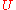. In an adiabatic process, adiabatic work takes the system from a reference state with internal energy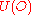to an arbitrary eventual one with internal energy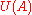: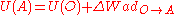where, following IUPAC convention we take as positive the work done on the system.

To go from a state A to a state B we can take a path that goes through the reference state, since the adiabatic work is independent of the path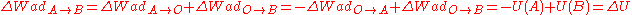### Isothermal diabatic processes

A complementary observable aspect of the first law is about heat transfer
Heat transfer
Heat transfer is a discipline of thermal engineering that concerns the exchange of thermal energy from one physical system to another. Heat transfer is classified into various mechanisms, such as heat conduction, convection, thermal radiation, and phase-change transfer...

.

When the system does not evolve adiabatically, it is observed that the work exerted on the system does not coincide with the increase in its internal energy, which, being a state function, can be used for both adiabatic and non-adiabatic processes.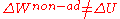The difference is due to the transfer of heat
Heat transfer
Heat transfer is a discipline of thermal engineering that concerns the exchange of thermal energy from one physical system to another. Heat transfer is classified into various mechanisms, such as heat conduction, convection, thermal radiation, and phase-change transfer...

into the system, and the process is called diabatic. Heat transfer can be measured by calorimetry
Calorimetry
Calorimetry is the science of measuring the heat of chemical reactions or physical changes. Calorimetry is performed with a calorimeter. The word calorimetry is derived from the Latin word calor, meaning heat...

.

If the system is at constant temperature during the heat transfer, the transfer is called isothermal diabatic, and we may write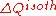.

Putting the two complementary aspects together, adiabatic and isothermal diabatic, the inequality can be transformed into an equality as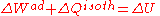This combined statement is the expression the first law of thermodynamics for finite processes composed of distinct adiabatic and isothermal diabatic segments.

In particular, if no work is exerted on a thermally isolated system we have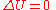.

This is one aspect of the law of conservation of energy and can be stated:
The internal energy of an isolated system remains constant.

## State functional formulation

The infinitesimal heat and work in the equations above are denoted by δ, rather than exact differential
Exact differential
A mathematical differential is said to be exact, as contrasted with an inexact differential, if it is of the form dQ, for some differentiable function Q....

s denoted by d, because they do not describe the state of any system. The integral of an inexact differential depends upon the particular path taken through the space of thermodynamic parameters while the integral of an exact differential depends only upon the initial and final states. If the initial and final states are the same, then the integral of an inexact differential may or may not be zero, but the integral of an exact differential will always be zero. The path taken by a thermodynamic system through a chemical or physical change is known as a thermodynamic process.

An expression of the first law can be written in terms of exact differentials by realizing that the work that a system does is, in case of a reversible process, equal to its pressure times the infinitesimal change in its volume. In other words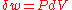where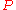is pressure
Pressure
Pressure is the force per unit area applied in a direction perpendicular to the surface of an object. Gauge pressure is the pressure relative to the local atmospheric or ambient pressure.- Definition :...

and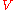is volume. Also, for a reversible process, the total amount of heat added to a system can be expressed as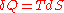where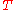is temperature
Temperature
Temperature is a physical property of matter that quantitatively expresses the common notions of hot and cold. Objects of low temperature are cold, while various degrees of higher temperatures are referred to as warm or hot...

and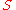is entropy
Entropy
Entropy is a thermodynamic property that can be used to determine the energy available for useful work in a thermodynamic process, such as in energy conversion devices, engines, or machines. Such devices can only be driven by convertible energy, and have a theoretical maximum efficiency when...

. Therefore, for a reversible process: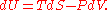Since U, S and V are thermodynamic functions of state, the above relation holds also for non-reversible changes. The above equation is known as the fundamental thermodynamic relation.

In the case where the number of particles in the system is not necessarily constant and may be of different types, the first law is written: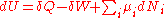where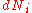is the (small) number of type-i particles added to the system, and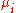is the amount of energy added to the system when one type-i particle is added, where the energy of that particle is such that the volume and entropy of the system remains unchanged.is known as the chemical potential
Chemical potential
Chemical potential, symbolized by μ, is a measure first described by the American engineer, chemist and mathematical physicist Josiah Willard Gibbs. It is the potential that a substance has to produce in order to alter a system...

of the type-i particles in the system. The statement of the first law, using exact differentials is now: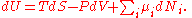If the system has more external variables than just the volume that can change, the fundamental thermodynamic relation generalizes to: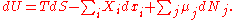Here theare the generalized forces corresponding to the external variables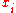.

A useful idea from mechanics is that the energy gained by a particle is equal to the force applied to the particle multiplied by the displacement of the particle while that force is applied. Now consider the first law without the heating term: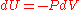. The pressure P  can be viewed as a force (and in fact has units of force per unit area) while dV  is the displacement (with units of distance times area). We may say, with respect to this work term, that a pressure difference forces a transfer of volume, and that the product of the two (work) is the amount of energy transferred out of the system as a result of the process. If one were to make this term negative then this would be the work done on the system.

It is useful to view the TdS  term in the same light: With respect to this heat term, a temperature difference forces a transfer of entropy, and the product of the two (heat) is the amount of energy transferred as a result of the process. Here, the temperature is known as a "generalized" force (rather than an actual mechanical force) and the entropy is a generalized displacement.

Similarly, a difference in chemical potential between groups of particles in the system forces a transfer of particles, and the corresponding product is the amount of energy transferred as a result of the process. For example, consider a system consisting of two phases: liquid water and water vapor. There is a generalized "force" of evaporation which drives water molecules out of the liquid. There is a generalized "force" of condensation which drives vapor molecules out of the vapor. Only when these two "forces" (or chemical potentials) are equal will there be equilibrium, and the net transfer will be zero.

The two thermodynamic parameters which form a generalized force-displacement pair are termed "conjugate variables". The two most familiar pairs are, of course, pressure-volume, and temperature-entropy.

## Spatially inhomogeneous systems

Classical thermodynamics is focused on homogeneous systems (e.g. Planck 1897/1903), which might be regarded as 'zero-dimensional' in the sense that they have no spatial variation. But it is desired to study also systems with distinct internal motion and spatial inhomogeneity. For such systems, the principle of conservation of energy is expressed in terms not only of internal energy as defined for homogeneous systems, but also in terms of kinetic and potential energies. How the total energy of a system is allocated between these three more specific kinds of energy varies according to the purposes of different writers; this is because these components of energy are to some extent mathematical artefacts rather than actually measured physical quantities. If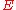denotes the total energy of a system, one may write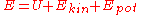where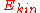and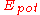denote respectively the kinetic and potential energies internal to the system.

Potential energy can be exchanged with the surroundings of the system when the surroundings impose a force field, such as gravitational or electromagnetic, on the system.

The distinction between internal and kinetic energy is hard to make in the presence of turbulent motion within the system, as friction gradually dissipates macroscopic kinetic energy of localised bulk flow into molecular random motion of molecules that is classified as internal energy. The rate of dissipation by friction of kinetic energy of localised bulk flow into internal energy, whether in turbulent or in streamlined flow, is an important quantity in non-equilibrium thermodynamics
Non-equilibrium thermodynamics
Non-equilibrium thermodynamics is a branch of thermodynamics that deals with systems that are not in thermodynamic equilibrium. Most systems found in nature are not in thermodynamic equilibrium; for they are changing or can be triggered to change over time, and are continuously and discontinuously...

. This is a serious difficulty for attempts to define entropy for time-varying spatially inhomogeneous systems.

## History

The discovery of the first law of thermodymanics was by way of many tries and mistakes of investigation, over a period of about half a century. The first full statements of the law were made by Clausius in 1850 as noted above, and by Rankine also in 1850; Rankine's statement was perhaps not quite as clear and distinct as was Clausius'. A main aspect of the struggle was to deal with the previously proposed caloric theory
Caloric theory
The caloric theory is an obsolete scientific theory that heat consists of a self-repellent fluid called caloric that flows from hotter bodies to colder bodies. Caloric was also thought of as a weightless gas that could pass in and out of pores in solids and liquids...

of heat.

Germain Hess in 1840 stated a conservation law
Hess's law
Hess' law is a relationship in physical chemistry named for Germain Hess, a Swiss-born Russian chemist and physician.The law states that the enthalpy change for a reaction that is carried out in a series of steps is equal to the sum of the enthalpy changes for the individual steps.The law is an...

for so-called 'heat of reaction' for chemical reactions, but this was not explicitly concerned with the relation between energy exchanges by heat and work.

According to Truesdell (1980), Julius Robert von Mayer
Julius Robert von Mayer
Julius Robert von Mayer was a German physician and physicist and one of the founders of thermodynamics...

in 1841 made a statement that meant that "in a process at constant pressure, the heat used to produce expansion is universally interconvertible with work", but this is not a general statement of the first law.

## See also

• Laws of thermodynamics
Laws of thermodynamics
The four laws of thermodynamics summarize its most important facts. They define fundamental physical quantities, such as temperature, energy, and entropy, in order to describe thermodynamic systems. They also describe the transfer of energy as heat and work in thermodynamic processes...

• Perpetual motion
Perpetual motion
Perpetual motion describes hypothetical machines that operate or produce useful work indefinitely and, more generally, hypothetical machines that produce more work or energy than they consume, whether they might operate indefinitely or not....

• Relativistic heat conduction
Relativistic heat conduction
The theory of relativistic heat conduction claims to be the only model for heat conduction that is compatible with the theory of special relativity, the second law of thermodynamics, electrodynamics, and quantum mechanics, simultaneously...

## External links

The source of this article is wikipedia, the free encyclopedia.  The text of this article is licensed under the GFDL.